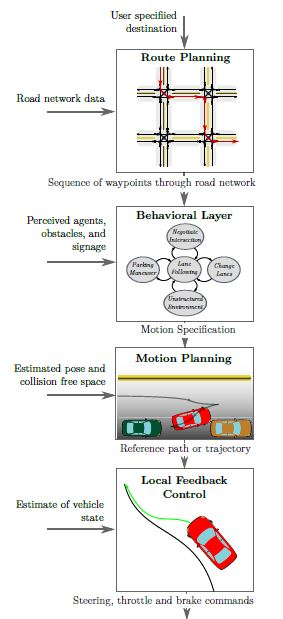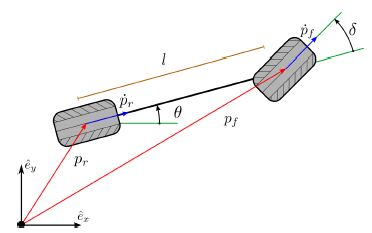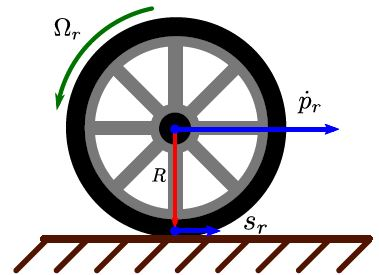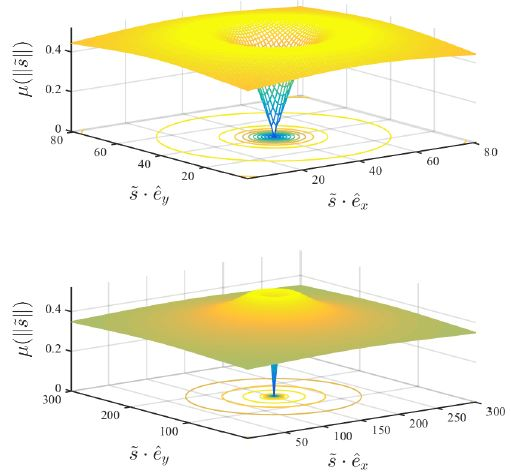# 城市道路自动驾驶车辆运动规划和控制技术综述

## I. 引言

本文主要集中于自动驾驶决策、运动规划和控制，尤其针对level3及以上系统。当代自动驾驶系统中的决策通常分层结构化为路线规划，行为决策制定，局部运动规划和反馈控制。然而，这些水平的划分相当模糊，文献中出现了这种方案的不同变化。 本文对提出的解决自动驾驶核心问题的方法进行了调查。 特别强调局部运动规划和控制的方法。
本文的其余部分结构如下：在第二部分中，介绍了决策过程层次结构的高级概述及其设计的一些方法。 第三部分回顾了用于近似城市环境中汽车移动性的模型，用于运动规划和反馈控制。 第四部分调查了有关运动规划的丰富文献，并讨论了它对自动驾驶汽车的适用性。 同样，第五节讨论了无人驾驶汽车的路径和轨迹稳定问题以及具体的反馈控制方法。 最后，第六节总结了关于最新技术和未来研究潜在领域的评论。

## II. 无人驾驶汽车中使用的决策层次概述

在本节中，我们将描述典型自动驾驶汽车的决策架构，并说明每个组件的功能。无人驾驶汽车本质上是自主决策系统，处理来自车载传感器的观测流，如雷达，激光雷达，照相机，GPS / INS单元和里程计。这些观察结果与关于道路网络的先验知识，道路规则，车辆动力学和传感器模型一起用于自动选择控制车辆运动的受控变量的值。智能车辆研究旨在尽可能地自动化驾驶任务。这个问题的常用方法是将感知和决策任务划分和组织成层次结构。感知系统使用先验信息和收集的观察数据来提供车辆状态及其周围环境的估计;然后，决策系统使用估计来控制车辆，从而完成驾驶目标。
典型的自动驾驶汽车的决策系统被分层地分解为四个部分（参见图II.1）：在最高级别，通过道路网络规划路线。 接下来是行为层，其决定将汽车推向目的地并遵守道路规则的局部驾驶任务。 然后，运动规划模块选择通过环境的连续路径以完成局部导航任务。然后，控制系统实时地校正执行规划运动中的错误。 在本节的其余部分，我们将更详细地讨论每个组件的功能。### A.路径规划

在最高级别，车辆的决策系统必须选择通过道路网络从其当前位置到所请求目的地的路线。通过将道路网络表示为具有与穿过道路段的成本相对应的边权的有向图，这样的路线可以被公式化为在道路网络图上找到最小成本路径的问题。然而，表示道路网络的图表可以包含数百万个边缘，使得诸如Dijkstra或A *之类的经典最短路径算法不切实际。交通网络中有效路线规划的问题引起了交通科学界的极大兴趣，导致一系列算法的发明，这些算法在一次性预处理步骤之后以毫秒为单位返回大陆规模网络上的最佳路线。全面调查和比较实用算法，可用于有效规划人类驾驶车辆和自动驾驶车辆的路线。

### B.行为决策

在找到路线计划之后，自主车辆必须能够导航所选择的路线并根据驾驶惯例和道路规则与其他交通参与者交互。 给定指定所选路线的一系列道路段，行为层负责基于其他交通参与者的感知行为，道路状况和来自基础设施的信号在任何时间点选择适当的驾驶行为。 例如，当车辆在交叉路口前到达停车线时，行为层将命令车辆停下来，观察交叉路口处其他车辆、自行车和行人的行为，并且一旦轮到他就让车辆行驶。
驾驶手册规定了特定驾驶环境的定性行为。 由于驾驶环境和行为都可以建模为有限集，因此自动化该决策的一种自然方法是将每个行为建模为有限状态机中的状态，其中过渡由感知的驾驶环境控制，例如相对于计划路线和附近车辆的相对位置。 事实上，DARPA城市挑战中的大多数团队采用有限状态机和特定于考虑驾驶场景的不同启发式机制作为行为控制机制。
然而，真实世界的驾驶，特别是在城市环境中，其特点是其他交通参与者的意图不确定。还研究了意图预测和估计其他车辆，自行车和行人未来轨迹的问题。所提出的解决方案技术为一类基于机器学习的技术（例如，高斯混合模型，高斯过程回归，据报道这些学习方法被用于谷歌的自动驾驶系统用于意图预测中），以及基于模型的方法（直接用于传感器测量估计意图）。
其他交通参与者行为的这种不确定性通常被考虑在行为层中，用于使用概率规划形式进行决策，例如马尔可夫决策过程（MDP）及其推广。例如，在MDP框架中制定了行为决策问题。 一些工作使用部分可观察的马尔可夫决策过程（POMDP）框架明确地模拟未观察到的驾驶场景和行人意图，并提出具体的近似解决方案策略。

### C.运动规划

当行为层决定要在当前环境中执行的驾驶行为时，其可以是例如巡航 - 车道，改变车道或右转，所选择的行为必须被转换成路径或轨迹，可由低级反馈控制器跟踪。 所产生的路径或轨迹必须满足车辆动力学约束的，对乘客来说是舒适的，并且避免与车载传感器检测到的障碍物的碰撞。寻找这样的路径或轨迹的任务是运动规划系统的责任。
自主车辆的运动规划任务对应于解决机器人文献中讨论的标准运动规划问题。 在大多数情况下，运动规划问题的精确解决方案在计算上是难以处理的。 因此，数值近似方法通常在实践中使用。在最流行的数值方法中，变分方法将问题视为函数空间中的非线性优化，图形搜索方法构建车辆状态空间的图形离散化并使用图形搜索测量方法搜索最短路径，此外基于树的增量方法，从车辆的初始状态逐步构造可到达状态的树，然后选择这种树的最佳分支。 第IV节更详细地讨论了与自动驾驶相关的运动规划方法。

### D.车辆控制

为了执行从运动规划系统中得到的参考路径或轨迹，使用反馈控制器来选择适当的驱动器输入以执行规划的运动并校正跟踪误差。 在执行规划运动过程中产生的跟踪误差部分地归因于车辆模型的不准确性。 因此，非常强调闭环系统的鲁棒性和稳定性。
已经提出了许多有效的反馈控制器来执行由运动规划系统提供的参考运动。 第五节详细讨论了相关技术的调查。

## III. 规划和控制建模

在本节中，我们将调查最常用的类车运动模型。 这些模型广泛用于控制和运动规划算法中，以响应于相关操作条件下的控制动作来近似车辆的行为。 高保真模型可以准确地反映车辆的响应，但是增加的细节可能使规划和控制问题复杂化。 这无法在所选模型的准确性与决策问题的难度之间进行权衡。 本节概述了一般建模概念以及用于运动规划和控制的模型调查。
建模从车辆构型的概念开始，表示其在世界中的姿势或位置。 例如，构型可以表示为汽车上一个点的平面坐标以及汽车的航向。 这是汽车构型空间的坐标系。 该坐标系描述了平面刚体运动（由二维特殊欧几里德群表示，SE（2）），并且是常用的构型空间。 然后必须规划和调节车辆运动以完成驾驶任务，同时遵守所选模型和控制引入的约束。

### A. 单边运动模型

在最基本的实际使用模型中，汽车由两个通过刚性连杆连接的轮子组成，并限制在一个平面内移动。 假设车轮在与地面的接触点处不会滑动，但可以绕其旋转轴线自由旋转。 前轮具有增加的自由度，允许其围绕垂直于运动平面的轴旋转。 这是模型转向。 这两个建模特征反映了大多数乘客在没有同时向前移动的情况下无法进行横向位移的经验。 更正式地说，对机动性的限制被称为非完整约束。 非完整约束表示为对汽车运动的微分约束。 该表达式根据坐标系的选择而变化。 该模型的变化被称为汽车式机器人，自行车模型，运动模型或单轨模型。
以下是用于构型的几个流行坐标系中的差分约束的推导。 参考图III.1，矢量${p}_{r}$$p_r$${p}_{f}$$p_f$表示后轮和前轮在具有基矢量$\left({\stackrel{^}{e}}_{x},{\stackrel{^}{e}}_{y},{\stackrel{^}{e}}_{z}\right)$$(\hat e_x,\hat e_y,\hat e_z)$的静止或惯性坐标系中的位置。 符号$\theta$$\theta$是描述车辆所面向的方向的角度。 这被定义为矢量${\stackrel{^}{e}}_{x}$$\hat e_x$${p}_{f}-{p}_{r}$$p_f - p_r$之间的角度。 对于由角度$\theta$$\theta$组成的坐标系，以及中的一个点${p}_{r}$$p_r$的运动，以及中的${p}_{f}$$p_f$，将导出微分约束。点${p}_{r}$$p_r$${p}_{f}$$p_f$的运动必须与车轮方向共线，以满足无滑动假设。表示为一个方程，后轮的约束为：

$\left({\stackrel{˙}{p}}_{r}\cdot {\stackrel{^}{e}}_{y}\right)\mathrm{cos}\left(\theta \right)-\left({\stackrel{˙}{p}}_{r}\cdot {\stackrel{^}{e}}_{x}\right)\mathrm{sin}\left(\theta \right)=0$

前轮约束为：

$\left({\stackrel{˙}{p}}_{f}\cdot {\stackrel{^}{e}}_{y}\right)\mathrm{cos}\left(\theta +\delta \right)-\left({\stackrel{˙}{p}}_{f}\cdot {\stackrel{^}{e}}_{x}\right)\mathrm{sin}\left(\theta +\delta \right)=0$

该表达式通常被改写为每一点沿基向量的分量运动形式。后轮沿${\stackrel{^}{e}}_{x}$$\hat e_x$方向的运动为：${x}_{r}={p}_{r}\cdot {\stackrel{^}{e}}_{x}$$x_r=p_r\cdot \hat e_x$；同理，沿${\stackrel{^}{e}}_{y}$$\hat e_y$方向的运动为：${y}_{r}={p}_{r}\cdot {\stackrel{^}{e}}_{y}$$y_r=p_r\cdot \hat e_y$。前进速度${v}_{r}={\stackrel{˙}{p}}_{r}\cdot \left({p}_{f}-{p}_{r}\right)/‖\left({p}_{f}-{p}_{r}\right)‖$$v_r=\dot p_r\cdot(p_f-p_r)/\|(p_f-p_r)\|$，即为${\stackrel{˙}{p}}_{r}$$\dot p_r$的大小，符号表明驾驶方向为前进或倒退。

$\begin{array}{rl}{\stackrel{˙}{x}}_{r}& ={v}_{r}\mathrm{cos}\left(\theta \right)\\ {\stackrel{˙}{y}}_{r}& ={v}_{r}\mathrm{sin}\left(\theta \right)\\ \stackrel{˙}{\theta }& =\frac{{v}_{r}}{l}\ast \mathrm{tan}\left(\delta \right)\end{array}$

或者，微分约束可以写为${p}_{f}$$p_f$的运动，

$\begin{array}{rl}{\stackrel{˙}{x}}_{f}& ={v}_{f}\mathrm{cos}\left(\theta +\delta \right)\\ {\stackrel{˙}{y}}_{f}& ={v}_{f}\mathrm{sin}\left(\theta +\delta \right)\\ \stackrel{˙}{\theta }& =\frac{{v}_{f}}{l}\ast \mathrm{tan}\left(\delta \right)\end{array}$

其中前轮的前进速度为${v}_{f}$$v_f$。前轮速度${v}_{f}$$v_f$与后轮速度之间的关系为：

$\frac{{v}_{r}}{{v}_{f}}=\mathrm{cos}\left(\delta \right)$

该模型的规划和控制问题涉及在车辆的机械极限内选择转向角$\delta \in \left[{\delta }_{min},{\delta }_{max}\right]$$\delta \in [\delta_{min},\delta_{max}]$,并且在可接受的范围内选择前进速度${v}_{r}\in \left[{v}_{min},{v}_{max}\right]$$v_r \in [v_{min},v_{max}]$
有时利用的简化，例如 ，是选择航向速度$\omega$$\omega$而不是转向角$\delta$$\delta$。这些量之间的关系可表示为

$\delta =\mathrm{arctan}\left(\frac{l\omega }{{v}_{r}}\right)$

简化正动力学为

$\stackrel{˙}{\theta }=\omega ,\phantom{\rule{1em}{0ex}}\omega \in \left[\frac{{v}_{r}}{l}\mathrm{tan}\left({\delta }_{min}\right),\frac{{v}_{r}}{l}\mathrm{tan}\left({\delta }_{max}\right)\right]$

该模型的一个重要变化是当${v}_{r}$$v_r$被修复时的情况。 这有时被称为Dubins汽车，在Lester Dubins之后，他们用规定的切线推导出最小时间运动。 另一个值得注意的变化是Reeds-Shepp汽车，当${v}_{r}$$v_r$采用单个前进和后退速度时，最小长度路径是已知的。 事实证明，这两种模型对运动规划具有一定的重要性，将在第四节进一步讨论。
运动学模型适用于规划低速路径（例如停车操纵和城市驾驶），其中惯性效应与无滑动假设所施加的移动性限制相比较小。 该模型的主要缺点是它允许瞬时转向角度变化，如果运动规划模块产生具有这种瞬时变化的解决方案，则这可能是有问题的。
通过增大（III.4）可以施加转向角的连续性，其中转向角积分了指令速率，如。式（III.4）变为

$\begin{array}{rl}{\stackrel{˙}{x}}_{f}& ={v}_{f}\mathrm{cos}\left(\theta +\delta \right)\\ {\stackrel{˙}{y}}_{f}& ={v}_{f}\mathrm{sin}\left(\theta +\delta \right)\\ \stackrel{˙}{\theta }& =\frac{{v}_{f}}{l}\ast \mathrm{tan}\left(\delta \right)\\ \stackrel{˙}{\delta }& ={v}_{\delta }\end{array}$

除了转向角的限制之外，现在可以限制转向速率:${v}_{\delta }\in \left[{\stackrel{˙}{\delta }}_{min},{\stackrel{˙}{\delta }}_{max}\right]$$v_{\delta}\in [\dot\delta_{min},\dot\delta_{max}]$。 汽车的速度${v}_{r}$$v_r$也会出现同样的问题，可以用同样的方法解决。 这种技术的缺点是模型的尺寸增加，这可能使运动规划和控制问题复杂化。
坐标系的选择不限于使用其中一个车轮位置作为位置坐标。 对于使用经典力学原理导出的模型，可以方便地使用质心作为，中的位置坐标，或，中的摆动中心。

### B.惯性效应

当车辆的加速度足够大时，轮胎与地面之间的无滑动假设变得无效。 在这种情况下，车辆的更精确模型是满足基本动量原理的刚体。 也就是说，加速度与轮胎上的地面产生的力成比例。 将${p}_{c}$$p_c$作为车辆质量中心和配置的坐标（参见图III.2），车辆的运动取决于：

$\begin{array}{rl}m{\stackrel{¨}{p}}_{c}& ={F}_{f}+{F}_{r}\\ {I}_{zz}\stackrel{¨}{\theta }& =\left({p}_{c}-{p}_{f}\right)×{F}_{f}+\left({p}_{c}-{p}_{f}\right)×{F}_{r}\end{array}$${F}_{r}$$F_r$${F}_{f}$$F_f$的表达式取决于建模假设，，，，但在任何情况下，表达式都可能很繁琐。 因此，等式（III.10） - （III.15）提供了详细的推导作为参考。
地面和轮胎之间的力被建模为取决于轮胎在地面上滑动的速率。 尽管质心用作构型的坐标，但是需要每个车轮相对于地面的速度来确定该相对速度。 这三点之间的运动关系是

$\begin{array}{rl}{p}_{r}& ={p}_{c}+\left(\begin{array}{c}-{l}_{r}\mathrm{cos}\left(\theta \right)\\ -{l}_{r}\mathrm{sin}\left(\theta \right)\\ 0\end{array}\right),\\ {\stackrel{˙}{p}}_{r}& ={\stackrel{˙}{p}}_{c}+\left(\begin{array}{c}0\\ 0\\ \stackrel{˙}{\theta }\end{array}\right)×\left(\begin{array}{c}-{l}_{r}\mathrm{cos}\left(\theta \right)\\ -{l}_{r}\mathrm{sin}\left(\theta \right)\\ 0\end{array}\right),\\ {p}_{f}& ={p}_{c}+\left(\begin{array}{c}{l}_{f}\mathrm{cos}\left(\theta \right)\\ {l}_{f}\mathrm{sin}\left(\theta \right)\\ 0\end{array}\right),\\ \\ {\stackrel{˙}{p}}_{f}& ={\stackrel{˙}{p}}_{c}+\left(\begin{array}{c}0\\ 0\\ \stackrel{˙}{\theta }\end{array}\right)×\left(\begin{array}{c}{l}_{r}\mathrm{cos}\left(\theta \right)\\ {l}_{f}\mathrm{sin}\left(\theta \right)\\ 0\end{array}\right)\end{array}$

这些运动学关系用于确定每个轮胎与地面接触的点${s}_{r},{s}_{f}$$s_r,s_f$的速度。这些点的速度称为轮胎滑动速度。通常，${s}_{r},{s}_{f}$$s_r,s_f$因车轮的角速度与${\stackrel{˙}{p}}_{r},{\stackrel{˙}{p}}_{f}$$\dot p_r,\dot p_f$不同。运动关系为：

$\begin{array}{rl}{s}_{r}& ={\stackrel{˙}{p}}_{r}+{\omega }_{r}×R\\ {s}_{f}& ={\stackrel{˙}{p}}_{f}+{\omega }_{f}×R\end{array}$

轮子的角速度通过下式给定：

${\omega }_{r}=\left(\begin{array}{c}{\mathrm{\Omega }}_{r}\mathrm{sin}\left(\theta \right)\\ -{\mathrm{\Omega }}_{r}\mathrm{cos}\left(\theta \right)\\ 0\end{array}\right),\phantom{\rule{1em}{0ex}}{\omega }_{f}=\left(\begin{array}{c}{\mathrm{\Omega }}_{f}\mathrm{sin}\left(\theta +\delta \right)\\ -{\mathrm{\Omega }}_{f}\mathrm{cos}\left(\theta +\delta \right)\\ 0\end{array}\right)$

其中$R=\left(0,0,-r{\right)}^{T}$$R=(0,0,-r)^T$.车辆半径为标量值$r$$r$,${\mathrm{\Omega }}_{\left\{r,f\right\}}$$\Omega_{\{r,f\}}$分别为每一个轮子相对于车子的角速度。后轮图例如图III.3所示。在静态条件下，或者当质心的高度可以近似为${p}_{c}\cdot {\stackrel{^}{e}}_{z}\approx 0$$p_c \cdot \hat e_z \approx 0$时，垂直于地面的力的分量${F}_{\left\{r,f\right\}}\cdot {\stackrel{^}{x}}_{z}$$F_{\{r,f\}}\cdot \hat x_z$可以通过静力 - 力矩平衡来计算：

${F}_{f}\cdot {\stackrel{^}{e}}_{z}=\frac{{l}_{r}mg}{{l}_{f}+{l}_{r}},\phantom{\rule{1em}{0ex}}{F}_{r}\cdot {\stackrel{^}{e}}_{z}=\frac{{l}_{f}mg}{{l}_{f}+{l}_{r}}.$

然后使用法向力来计算每个轮胎上的牵引力以及滑动和轮胎行为的摩擦系数模型$\mu$$\mu$。 后轮胎上的牵引力以分量形式给出：

$\begin{array}{r}{F}_{r}\cdot {\stackrel{^}{e}}_{x}=-\frac{{F}_{r}\cdot {\stackrel{^}{e}}_{z}\mu \left(\frac{‖{s}_{r}‖}{{\mathrm{\Omega }}_{r}r}\right){s}_{r}}{‖{s}_{r}‖}\cdot {\stackrel{^}{e}}^{x},\\ {F}_{r}\cdot {\stackrel{^}{e}}_{y}=-\frac{{F}_{r}\cdot {\stackrel{^}{e}}_{z}\mu \left(\frac{‖{s}_{r}‖}{{\mathrm{\Omega }}_{r}r}\right){s}_{r}}{‖{s}_{r}‖}\cdot {\stackrel{^}{e}}^{y}\end{array}$

相同的表达式描述了前轮胎，其下标$r$$r$由下标$f$$f$代替。 上面的公式将牵引力建模为与滑差反平行，其大小与法向力成比例，并且与滑移率（后部${\mathrm{\Omega }}_{r}r$$\Omega_r r$和前部${\mathrm{\Omega }}_{f}r$$\Omega_f r$单位化的滑动的大小）非线性相关。 组合（III.10） - （III.15）根据控制变量，广义坐标和它们的速度得出汽车每个车轮上的净力的表达式。 公式（III.14），以及$\mu$$\mu$的以下模型：

$\mu \left(\frac{‖{s}_{r}‖}{{\mathrm{\Omega }}_{r}r}\right)=D\mathrm{sin}\left(C\mathrm{arctan}\left(B\frac{‖{s}_{r}‖}{{\mathrm{\Omega }}_{r}r}\right)\right)$

（III.14）的旋转对称性与（III.15）中的峰值一起导致轮胎可以在任何方向上施加的最大标准力。 该峰值被称为图III.4中所示的摩擦圆。本节中讨论的模型经常出现在关于无人驾驶汽车的运动规划和控制的文献中。 它们适用于本调查中讨论的运动规划和控制任务。 然而，诸如电子稳定性控制和主动悬架系统的较低级别控制任务通常使用更复杂的车辆，转向和传动系统模型。

©️2019 CSDN 皮肤主题: 大白 设计师: CSDN官方博客